JEE  >  VITEEE PCME Mock Test - 5

# VITEEE PCME Mock Test - 5

Test Description

## 125 Questions MCQ Test VITEEE: Subject Wise and Full Length MOCK Tests | VITEEE PCME Mock Test - 5

VITEEE PCME Mock Test - 5 for JEE 2023 is part of VITEEE: Subject Wise and Full Length MOCK Tests preparation. The VITEEE PCME Mock Test - 5 questions and answers have been prepared according to the JEE exam syllabus.The VITEEE PCME Mock Test - 5 MCQs are made for JEE 2023 Exam. Find important definitions, questions, notes, meanings, examples, exercises, MCQs and online tests for VITEEE PCME Mock Test - 5 below.
Solutions of VITEEE PCME Mock Test - 5 questions in English are available as part of our VITEEE: Subject Wise and Full Length MOCK Tests for JEE & VITEEE PCME Mock Test - 5 solutions in Hindi for VITEEE: Subject Wise and Full Length MOCK Tests course. Download more important topics, notes, lectures and mock test series for JEE Exam by signing up for free. Attempt VITEEE PCME Mock Test - 5 | 125 questions in 150 minutes | Mock test for JEE preparation | Free important questions MCQ to study VITEEE: Subject Wise and Full Length MOCK Tests for JEE Exam | Download free PDF with solutions
 1 Crore+ students have signed up on EduRev. Have you?
VITEEE PCME Mock Test - 5 - Question 1

### The rate of change of the volume of a sphere w.r.t. its surface area, when the radius is 2cm, is

VITEEE PCME Mock Test - 5 - Question 2

### A real value of x will satisfy the equation [((3 - 4 i x)/( 3 + 4i x))] = α - iβ ( α ,β real),if

VITEEE PCME Mock Test - 5 - Question 3

###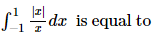VITEEE PCME Mock Test - 5 - Question 4

The area bounded by the curve y = (x + 1)2, y = (x - 1)2 and the line y = 1/4 is

Detailed Solution for VITEEE PCME Mock Test - 5 - Question 4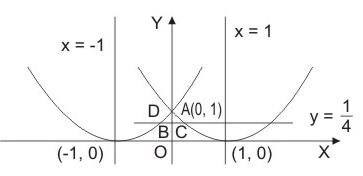Given curves are
(x + 1)2 = y .....(1)
and (x - 1)2 = y .....(2)
Axis of parabola (1) is x = - 1 and vertex is (- 1, 0) and it meets y-axis at (0,1)
Axis of parabola (2) is x = 1 and vertex is (1, 0) and it cuts y - axis at (0, 1)
When y = 1/4
from (2), x - 1 =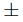1/2
∴ x = 1/2 , 3/2
∴ C ≡ ( 1/2 , 1/4 )
Required area = 2 area BACB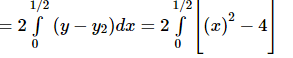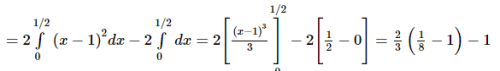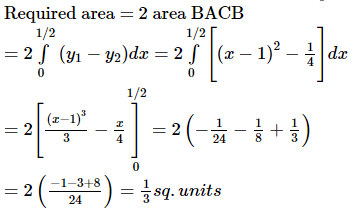VITEEE PCME Mock Test - 5 - Question 5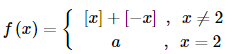where [x] denotes the greatest integer less than or equal to x, is continuous at x = 2, then the value of a is equal to

VITEEE PCME Mock Test - 5 - Question 6

All the roots of the equation zn cos θo + zn − 1 cos θ1 + … + cos θn = 2 , where θo , θ1 , θ2 , … , θn ∈ R will satisfy

VITEEE PCME Mock Test - 5 - Question 7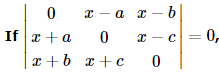, then the value of x is

VITEEE PCME Mock Test - 5 - Question 8

If f(x)   = tanx/x , x ≠ 0 ; f x = 1, x = 0 , then the function at x = 0 is

VITEEE PCME Mock Test - 5 - Question 9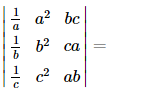Detailed Solution for VITEEE PCME Mock Test - 5 - Question 9

Multiply R1, R2, R3 by a, b, c respectively and divide by abc
Take abc common from C3 so that C1, C3 are identical

VITEEE PCME Mock Test - 5 - Question 10

A solution of the differential equation x (dy/dx)2 + (y-x) dy/dx - y = 0 is

VITEEE PCME Mock Test - 5 - Question 11

y = A ex + B e2x  + C e3x  satisfies the diferential equation

VITEEE PCME Mock Test - 5 - Question 12

The solution of differential equation (dy/dx)=[(1+y2)/(1+x2)] is

VITEEE PCME Mock Test - 5 - Question 13

Which equation has the solution y=A sinx+B cosx?

VITEEE PCME Mock Test - 5 - Question 14

If y=log logx, ey(dy/dx)=

VITEEE PCME Mock Test - 5 - Question 15

If f : A → B is a bijective function then f-1 ο f=

VITEEE PCME Mock Test - 5 - Question 16

∫ [sin(logx) + cos(logx)] dx =

VITEEE PCME Mock Test - 5 - Question 17

∫x cos x dx =

VITEEE PCME Mock Test - 5 - Question 18

If tan-1 x + tan-1 y + tan-1 z = π/2 , then 1 - xy - yz - zx =

Detailed Solution for VITEEE PCME Mock Test - 5 - Question 18

Let tan-1 x = A, tan-1 y = B, tan-1 z = C,
Then x = tan A, y = tan B, z = tan C, A + B + C = π/2
A + B + C = π/2
⇒ tan (A + B + C) is not defined
⇒ 1 - S2 = 0
⇒ 1 - tan A tan B - tan B tan C - tan C tan A = 0
⇒ 1 - xy - yz - zx = 0

VITEEE PCME Mock Test - 5 - Question 19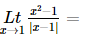VITEEE PCME Mock Test - 5 - Question 20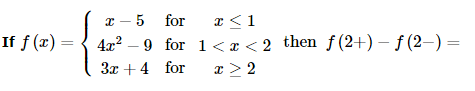VITEEE PCME Mock Test - 5 - Question 21

A and B are square matrices of the same order. Which is correct?

VITEEE PCME Mock Test - 5 - Question 22

"It has two pairs of opposite sides parallel." Which of the following make this open sentence true?

Detailed Solution for VITEEE PCME Mock Test - 5 - Question 22

Parallelograms and rectangles have two pairs (sets) of parallel sides. A trapezoid has one pair (set) of parallel sides.

VITEEE PCME Mock Test - 5 - Question 23

If A,B,C are matrices of order nxn then (ABC)'=

VITEEE PCME Mock Test - 5 - Question 24

A parallelogram is cut by two sets of m lines parallel to the sides, the number of parallelogram thus formed is

VITEEE PCME Mock Test - 5 - Question 25

The chance of throwing a total of 7 or 12 with 2 dice, is

VITEEE PCME Mock Test - 5 - Question 26

From a box containing 10 cards, numbered 1, 2,3,......,10. Four cards are drawn together, what is the probability of their sum is even ?

VITEEE PCME Mock Test - 5 - Question 27

The probabilities that three men hit a target are 1/6 , 1/4 , 1/3 respectively. Each shoots once the target what is the probability that exactly one of the them hits the target?

Detailed Solution for VITEEE PCME Mock Test - 5 - Question 27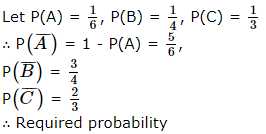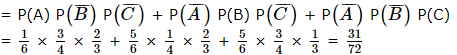VITEEE PCME Mock Test - 5 - Question 28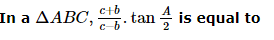Detailed Solution for VITEEE PCME Mock Test - 5 - Question 28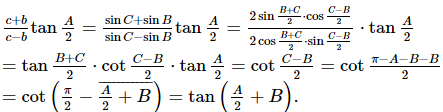VITEEE PCME Mock Test - 5 - Question 29

In the expansion of (1 + x)n,coefficients of 2nd ,3rd and 4th terms are in A.P.Then n is equal to

VITEEE PCME Mock Test - 5 - Question 30

The mean deviation of the numbers 3, 4, 5, 6, 7 is

VITEEE PCME Mock Test - 5 - Question 31

The mean age of a combined group of men and women is 30 years. If the means of the age of men and women are respectively 32 and 27, then the percentage of women in the group is

VITEEE PCME Mock Test - 5 - Question 32

If equations x = cy + bz, y = az + cx and z = bx + ay have any solution except x = 0, y = 0, z = 0, then the relation between a, b and c is

Detailed Solution for VITEEE PCME Mock Test - 5 - Question 32

The system of homogeneous equations-
x − cy − bz = 0......(i)
cx − y - bz = 0
bx - ay − z = 0
has non-trivial solution (since x, y, z are not all zero)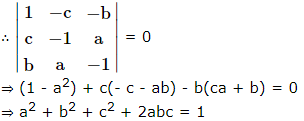VITEEE PCME Mock Test - 5 - Question 33

The value of x2 + 2bx + c is positive if

Detailed Solution for VITEEE PCME Mock Test - 5 - Question 33

The given expression be
x2 + 2bx + c
Comparing it with Ax2 + Bx + c, we get
A = 1, B = 2b, C = c
Also A = 1 > 0
x2 + 2bx + c will be positive i.e.
will be of the same sign as that of A,
if B2 - 4AC < 0
4b2 - 4(1) (c) < 0
or b2 < c

VITEEE PCME Mock Test - 5 - Question 34

The equation of the plane through intersection of planes x + 2y + 3z = 4 and 2x + y - z = -5 and perpendicular to the plane 5x + 3y + 6z + 8 = 0 is

VITEEE PCME Mock Test - 5 - Question 35

The radius of the sphere which passes thro' the points (0,0,0),(1,0,0),(0,1,0) and (0,0,1) is

VITEEE PCME Mock Test - 5 - Question 36

If 2sec2α = tanβ + cotβ, then one of the value of (α+β) is

VITEEE PCME Mock Test - 5 - Question 37

The number of tangents to the circle x2 + y2 - 2x + 4y = 0 through the point (-1, 2) is

VITEEE PCME Mock Test - 5 - Question 38

If a circle passes through the point (a, b) and cuts the cirlce x2+ y2 = p2 orthogonally, then the equation of the locus of its centre is

VITEEE PCME Mock Test - 5 - Question 39

If vectors i-3j+2k, -i+2j represent the diagonals of a parallelogram, then its area is

VITEEE PCME Mock Test - 5 - Question 40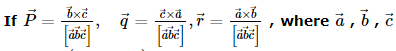are three non coplanar vectors, then the value of the expression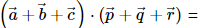VITEEE PCME Mock Test - 5 - Question 41
An A.C. source is connected to a resistance circuit. Then
VITEEE PCME Mock Test - 5 - Question 42
To a charged particle, which is moving with a constant initial velocity $\stackrel{\to }{V}$, a uniform magnetic field is applied in the direction of velocity; then
VITEEE PCME Mock Test - 5 - Question 43

Light of wave length λ and photon energy 2eV falling on a metal surface produces photoelectrons of maximum velocity V. If the λ is decreased by 25% and the maximum velocity of electron is doubled, the work function of the metal is (in eV)

VITEEE PCME Mock Test - 5 - Question 44
Of the following, the one that does not make use of chemical effect of electric current is
VITEEE PCME Mock Test - 5 - Question 45
Consider telecommunication through optical fibres. Which of the following statements is not correct
VITEEE PCME Mock Test - 5 - Question 46
An insulator plate is passed between the plates of a capacitor. Then current
VITEEE PCME Mock Test - 5 - Question 47
Four identical cells each having an electromotive force (e.m.f.) of 12V, are connected in parallel. The resultant electromotive force (e.m.f.) of the combination is
VITEEE PCME Mock Test - 5 - Question 48
An electrical meter of internal resistance 20$Ω$ gives a full scale deflection when one milliampere current flows through it. The maximum current, that can be measured by using three resistors of resistance 12$Ω$ each, in milliamperes is
VITEEE PCME Mock Test - 5 - Question 49
Two identical cells of the same e.m.f. and same internal resistance give the same current through an external resistance 2 Ω, regardless of whether they are connected in series or parallel. The internal resistance of the cell is
VITEEE PCME Mock Test - 5 - Question 50
Two wires of the same material are given. The first wire is twice as long as the second and has twice the diameter of the second. The resistance of the first will be
VITEEE PCME Mock Test - 5 - Question 51

Which of the following is true for photon

VITEEE PCME Mock Test - 5 - Question 52
A choke coil has
VITEEE PCME Mock Test - 5 - Question 53
In an ideal inductor, L =4 H and ω=100 rad/s. The power developed is
VITEEE PCME Mock Test - 5 - Question 54
The energy stored in coil carrying current I s U. If current is halved, then energy stored in the coil will be
VITEEE PCME Mock Test - 5 - Question 55
Which is having minimum wavelength:
VITEEE PCME Mock Test - 5 - Question 56

If the electric flux entering and leaving an enclosed surface respectively is φ1 and φ2  , the electric charge inside the surface will be

Detailed Solution for VITEEE PCME Mock Test - 5 - Question 56

Hint: Here, we have to apply gauss's law to find electric charge.

Solution:

We know that, electric flux ϕ1​ (or electric field lines) entering in a closed surface is -ve and electric flux ϕ2​ (or electric field lines) leaving a closed surface is +ve.

Hence, net electric flux through the closed surface,

ϕ=ϕ2​−ϕ1​

Now, according to Gauss' theorem, the net electric flux ϕ passing through a closed surface is equal to the 1/ε0​ times of the total charge q, inside the surface.

Step1: Apply gauss's law

Given, Net electric flux, ϕ=(ϕ2​−ϕ1​)

ϕ=ε0​qin​​

⇒qin​=ε0​ϕ

∴qin​=(ϕ2​−ϕ1​)ε0​

VITEEE PCME Mock Test - 5 - Question 57
Two charges -10 C and + 10 C are placed 10cm apart. Potential at the centre of the line joining two charges is
VITEEE PCME Mock Test - 5 - Question 58

n small drops of same size are charged to V volt each. They coalesce to form a bigger drop. The potential of the bigger drop is

VITEEE PCME Mock Test - 5 - Question 59
In C.G.S system the capacity of a spherical conductor is numerically equal to its
VITEEE PCME Mock Test - 5 - Question 60

A coil of n number of turns is wound tightly in the form of a spiral with inner and outer radii a and b respectively. When a current of strength I is passed through the coil, the magnetic field at its centre is

VITEEE PCME Mock Test - 5 - Question 61

If 1000 droplets each of charge q and radius r are mixed to form a big drop, then the potential of big drop, as compared to small droplet will be

Detailed Solution for VITEEE PCME Mock Test - 5 - Question 61

If the value of potential on each small drop be V and potential on each small drop be V '
V ' = n 2/3 V
⇒ V ' = 1000 2/3 V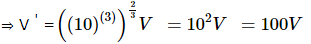VITEEE PCME Mock Test - 5 - Question 62

The masses of neutron, proton and deuteron in a.m.u are 1.00893 1.00813 and 2.01473 respectively. The packing fraction of the deuteron in a.m.u is

Detailed Solution for VITEEE PCME Mock Test - 5 - Question 62

Packing fraction = (M - A)/A , where M is the atomic mass and A is the mass number.
P = M - A/A = (2.01473 - 2) /2 = 73.6 x 10-4

VITEEE PCME Mock Test - 5 - Question 63
The nucleus finally formed in fusion of protons in proton-proton cycle is
VITEEE PCME Mock Test - 5 - Question 64
Which is not electromagnetic wave in the following?
VITEEE PCME Mock Test - 5 - Question 65
Graph of maximum kinetic energy of the photoelectrons against ν, the frequency of the incident radiation on the metal, is a straight line of slope equal to
VITEEE PCME Mock Test - 5 - Question 66
Light of wavelength 4000Å is incident on a sodium surface for which the threshold wave length of photo-electrons is 5420Å. The work function of sodium is
VITEEE PCME Mock Test - 5 - Question 67

Capacitance of a capacitor becomes 7/6 times its original value if a dielectric slab of thickness, t = 2/3 d is introduced in between the plates. 'd' is the separation between the plates. The dielectric constant of the dielectric slab is

Detailed Solution for VITEEE PCME Mock Test - 5 - Question 67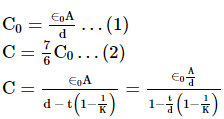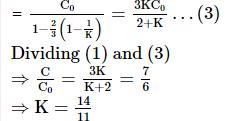VITEEE PCME Mock Test - 5 - Question 68

A ray of light is incident on a plane mirror at an angle of 600 . The angle of deviation produced by the mirror is

VITEEE PCME Mock Test - 5 - Question 69
In order to increase the angular magnification of a simple microscope, one should increase
VITEEE PCME Mock Test - 5 - Question 70
In forward bias, in a p - n junction the potential barrier
VITEEE PCME Mock Test - 5 - Question 71
Transistor can be used as
VITEEE PCME Mock Test - 5 - Question 72

For a transistor the parameter β = 99. The value of the parameter α is

VITEEE PCME Mock Test - 5 - Question 73
In extrinsic semiconductors
VITEEE PCME Mock Test - 5 - Question 74
Bonding in a germanium crystal (semiconductor) is
VITEEE PCME Mock Test - 5 - Question 75
The cause of the potential barrier in a p-n diode is
VITEEE PCME Mock Test - 5 - Question 76

At 00K, fermi level for metals

VITEEE PCME Mock Test - 5 - Question 77

In Young's double slit experiment, the width of the fringes obtained with light of wavelength 6000 Angstrom is 2.0mm. The fringe width, if the entire apparatus is immersed in a liquid of refractive index 1.33, will be

Detailed Solution for VITEEE PCME Mock Test - 5 - Question 77

Fringe width in Young's double slit experiment β = Dλ/d
When apparatus is immersed in liquid, only wavelength of light (λ) changes
Wavelength of light is liquid,
λ' = λ/n , where n = refractive index of medium
Initial fringe width, β = Dλ/d .....(i)
Fringe width in liquid, β' = Dλ'/d .....(ii)
Dividing Equation (ii) by Equation (i), we get
β/ β = λ'/λ
or β'/β = λ n/λ = 1/n
or β' = β/n
The new fringe width, β' = 2.0/1.33 mm = 1.5 mm

VITEEE PCME Mock Test - 5 - Question 78
What is the effect on the interference fringes in a Young's double slit experiment, if the monochromatic source is replaced by another monochromatic source of shorter wavelength?
VITEEE PCME Mock Test - 5 - Question 79

In the propagation of light waves, the angle between the direction of vibration and plane of polarisation is

VITEEE PCME Mock Test - 5 - Question 80
Both light and sound waves produce diffraction. It is more difficult to observe the diffraction with light waves because
VITEEE PCME Mock Test - 5 - Question 81

Acetaldehyde reacts with

VITEEE PCME Mock Test - 5 - Question 82

Glycerol on heating with potassium bisulphate yields

VITEEE PCME Mock Test - 5 - Question 83

In Cannizzaro's reaction

VITEEE PCME Mock Test - 5 - Question 84

Which of the following method is most appropriate for the manufacture of methane?

VITEEE PCME Mock Test - 5 - Question 85

Which of the following does not turn Schiff's reagent to pink?

VITEEE PCME Mock Test - 5 - Question 86

Ozonolysis of C₇H₁₄ gave 2-methyl-3-pentanone toluene. The alkene is

VITEEE PCME Mock Test - 5 - Question 87

Which of the following is an electrophilic reagent?

VITEEE PCME Mock Test - 5 - Question 88

The reaction, CH₂ = CH-CH₃ + HBr → CH₃CHBr - CH₃ is

VITEEE PCME Mock Test - 5 - Question 89

The energy stored in the cells of a living body is in the form of

VITEEE PCME Mock Test - 5 - Question 90

VITEEE PCME Mock Test - 5 - Question 91

Which of the following substance when boiled with caustic soda evolve ammonia ?

VITEEE PCME Mock Test - 5 - Question 92

To become a carbohydrate a compound must contain at least

VITEEE PCME Mock Test - 5 - Question 93

Which salt can be produced by the reaction of carbon monoxide and caustic soda (NaOH)?

VITEEE PCME Mock Test - 5 - Question 94

The acid showing salt like character in aqueous solutions is

VITEEE PCME Mock Test - 5 - Question 95

A chemical reaction is catalyzed by a catalyst X. Hence X

VITEEE PCME Mock Test - 5 - Question 96

A glass bulb is filled with NO₂ gas and immersed in an ice bath at 0oC which becomes colourless after some time . This colourless gas will be

VITEEE PCME Mock Test - 5 - Question 97

Plants and living beings are the examples of

VITEEE PCME Mock Test - 5 - Question 98

Medicine which is an antibiotic is

VITEEE PCME Mock Test - 5 - Question 99

In a closed insulated container a liquid is stirred with a paddle to increase the temperature. Which of the following is true

VITEEE PCME Mock Test - 5 - Question 100

The compound having highest dipole moment is

VITEEE PCME Mock Test - 5 - Question 101

Identify the reaction in which the heat liberated corresponds to the heat of formation ( Δ H )

VITEEE PCME Mock Test - 5 - Question 102

[Cr(H₂O)₆]Cl₃(at no. of Cr=24) has a magnetic moment of 3.83 BM. The correct distribution of 3d electrons in the Chromium of the complex is

VITEEE PCME Mock Test - 5 - Question 103

Which of the following is produced by reaction of RCN in sodium and alcohol ?

VITEEE PCME Mock Test - 5 - Question 104

The indicator that is obtained by coupling the diazonium salt of sulphanilic acid with N,N-dimethylaniline is

VITEEE PCME Mock Test - 5 - Question 105

The correct structure of Fe(CO)₅ is (Z = 26 for Fe)

VITEEE PCME Mock Test - 5 - Question 106

The cell potential of the following cell at 25 0C (in volts) is
Pt , H2 (1 atm) | H+ 0.01 M ∥ Cu2+ (0.1 M) | Cu (Eo Cu2+ ∕ Cu = + 0.337 V)

VITEEE PCME Mock Test - 5 - Question 107

A smuggler could not carry gold by chemically depositing iron on the gold surface since

VITEEE PCME Mock Test - 5 - Question 108

The oxidation no. of nitrogen in NaNO₂ is

Detailed Solution for VITEEE PCME Mock Test - 5 - Question 108

The formal oxidation state of the nitrogen atom in a nitrite is +3. This means that it is can be either oxidised to oxidation states +4 and +5 or reduced to oxidation states as low as -3.

VITEEE PCME Mock Test - 5 - Question 109

Microcosmic salt is

VITEEE PCME Mock Test - 5 - Question 110

Ether which is liquid at room temperature is

VITEEE PCME Mock Test - 5 - Question 111

A container contains 1 mole of a gas at 1 atm pressure and 27oC, and its volume is 24.6 ltres. If pressure is 10 atm and temperature 327oC,  then new volume is approximately

VITEEE PCME Mock Test - 5 - Question 112

VITEEE PCME Mock Test - 5 - Question 113

P4O10 has short and long P-O bonds. The number of short P-O bonds in this compound is

Detailed Solution for VITEEE PCME Mock Test - 5 - Question 113

P4O10 has four P = O bonds which are shorter than P-O single bonds.
Each P atom has three P-O single bonds and one P = O bond, i.e.,
a total of four P-O linkages

VITEEE PCME Mock Test - 5 - Question 114

Oxidation state of Fe in Fe₃O₄ is

VITEEE PCME Mock Test - 5 - Question 115

Pick up the correct statement

VITEEE PCME Mock Test - 5 - Question 116

Which of the following is least basic ?

VITEEE PCME Mock Test - 5 - Question 117

The isomers are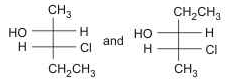VITEEE PCME Mock Test - 5 - Question 118

The catalytic activity of the transition metals and their compounds is ascribed to

VITEEE PCME Mock Test - 5 - Question 119

The separation of colloidal particles from those of molecular dimensions is called

VITEEE PCME Mock Test - 5 - Question 120

The brown ring complex compounds is formulated as [Fe(H2O)5NO] SO4. The oxidation state of iron is

VITEEE PCME Mock Test - 5 - Question 121

Find the Antonym of TIMID

VITEEE PCME Mock Test - 5 - Question 122

Find the Syonym of AFFLUENT

VITEEE PCME Mock Test - 5 - Question 123

Fill in the blank with appropriate word.
The Hubble Space Telescope will search for planets around other stars, a key to the..........for extra terrestrial life, and examine interstellar dust gases out of which stars are born.

VITEEE PCME Mock Test - 5 - Question 124

Out of the given alternatives, choose the one which can be substituted for the given capitalised word.

The staff have felt ON EDGE since they heard the rumour about retrenchment.

VITEEE PCME Mock Test - 5 - Question 125

Improve the sentence by choosing best alternative for capitalised part of the sentence.

It was indeed a shock for her, but she has LATER recovered from it.

## VITEEE: Subject Wise and Full Length MOCK Tests

1 videos|3 docs|73 tests
 Use Code STAYHOME200 and get INR 200 additional OFF Use Coupon Code
Information about VITEEE PCME Mock Test - 5 Page
In this test you can find the Exam questions for VITEEE PCME Mock Test - 5 solved & explained in the simplest way possible. Besides giving Questions and answers for VITEEE PCME Mock Test - 5, EduRev gives you an ample number of Online tests for practice

## VITEEE: Subject Wise and Full Length MOCK Tests

1 videos|3 docs|73 tests